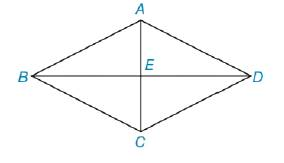Chapter 3.1, Problem 37E### Elementary Geometry for College St...

6th Edition
Daniel C. Alexander + 1 other
ISBN: 9781285195698

#### Solutions

Chapter
Section### Elementary Geometry for College St...

6th Edition
Daniel C. Alexander + 1 other
ISBN: 9781285195698
Textbook Problem
1 views

# In quadrilateral ABCD, A C ¯ and B D ¯ are perpendicular bisectors of each other. Name all triangles that are congruent to: a) Δ A B E b) Δ A B C c) Δ A B DTo determine

a)

To find:

The name of the triangle is congruent to ΔABE.

Explanation

Procedure used:

The perpendicular bisectors, bisect each other equally and makes right angles.

Given:

The quadrilaterals ABCD is shown in the figure below,

Figure (1)

From figure (1),

AC¯ and BD¯ are perpendicular bisectors of each other.

Calculation:

Consider the triangles,

Since, AC¯ and BD¯ are perpendicular bisectors to each other. Then,

The side BE¯ and the side DE¯ are equal to each other. So,

BE¯DE¯

The side AE¯ and the side CE¯ are equal to each other. So,

AE¯CE¯

And, the angle AEB, the angle AED, the angle DEC and the angle BEC makes right angle, so

To determine

b)

The triangle ΔABC is congruent to the triangle.

To determine

c)

The triangle ΔABD is congruent to the triangle.

### Still sussing out bartleby?

Check out a sample textbook solution.

See a sample solution

#### The Solution to Your Study Problems

Bartleby provides explanations to thousands of textbook problems written by our experts, many with advanced degrees!

Get Started

#### In Exercises 1124, find the indicated limits, if they exist. 21. limxx2x21

Applied Calculus for the Managerial, Life, and Social Sciences: A Brief Approach

#### In the problems 7-8, find the intercepts and graph the functions 7x+5y=21

Mathematical Applications for the Management, Life, and Social Sciences

#### Sometimes, Always, or Never: The sum of two elementary functions is an elementary function.

Study Guide for Stewart's Single Variable Calculus: Early Transcendentals, 8th